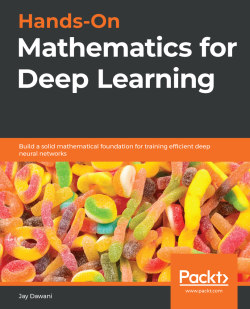# Hands-On Mathematics for Deep Learning4.8 (6 reviews total)
By Jay Dawani
• \$28.99 eBook
• \$41.99 Print + eBook
What do you get with a Packt Subscription?

• Constantly updated with 100+ new titles each month
• Breadth and depth in over 1,000+ technologies

Most programmers and data scientists struggle with mathematics, having either overlooked or forgotten core mathematical concepts. This book uses Python libraries to help you understand the math required to build deep learning (DL) models.

You'll begin by learning about core mathematical and modern computational techniques used to design and implement DL algorithms. This book will cover essential topics, such as linear algebra, eigenvalues and eigenvectors, the singular value decomposition concept, and gradient algorithms, to help you understand how to train deep neural networks. Later chapters focus on important neural networks, such as the linear neural network and multilayer perceptrons, with a primary focus on helping you learn how each model works. As you advance, you will delve into the math used for regularization, multi-layered DL, forward propagation, optimization, and backpropagation techniques to understand what it takes to build full-fledged DL models. Finally, you’ll explore CNN, recurrent neural network (RNN), and GAN models and their application.

By the end of this book, you'll have built a strong foundation in neural networks and DL mathematical concepts, which will help you to confidently research and build custom models in DL.

Publication date:
June 2020
Publisher
Packt
Pages
364
ISBN
9781838647292

# Section 1: Essential Mathematics for Deep Learning

In this section, you will learn how to use the core mathematical and modern computational techniques that are used to design and implement deep learning algorithms.

This section is comprised of the following chapters: